# Normal Probability Plots

I have a confession to make.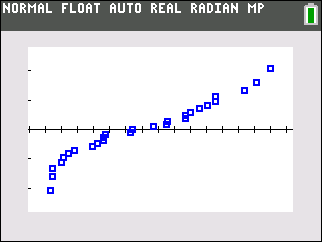When I first started teaching AP Statistics in 2005, I had no idea why a Normal probability plot (an example is shown to the left) was important... or what it told us about data. I busy trying to stay a day ahead of students that first year. I never really sat down with several textbooks to compare definitions and examples as I probably should have. Simply put, when students asked, I told them the canned answer: "The more linear the plot is, the more "Normal" the data is." We'd use the calculator to make the plot, look at it, and move on.

Let's take a closer look at why we study a Normal probability plot in AP Statistics. I will do some borrowing from various discussion board posts of the past on the AP Stats forum and will add some commentary as we go.

First, consider the method we use to compute a z-score; that is, a positional score for Normally distributed data that indicates the number of standard deviation units above or below the mean a particular data point lives. For example, if z = -1.2, then the data point is 1.2 standard deviations below the mean. It makes sense that a standardized score [ z = (x-μ)/σ] depends on two things: the data value's physical distance from the mean *and* the distance tempered by a measure of spread, specifically the standard deviation. Let's isolate x in this equation to see what happens.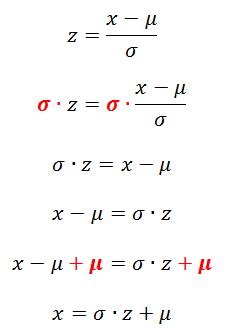The algebra above is commonly used in problems where we are asked to find a score which corresponds to a particular percentile rank. For example, if the mean score of the ACT is 18, and the standard deviation is 6, then what composite score puts a student in the 70th percentile of all test takers that day? A score slightly north of 21, as shown below.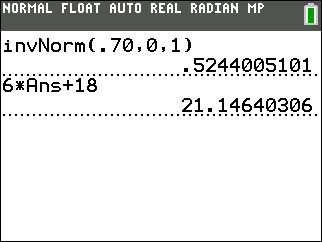The InvNorm command above finds the z-score corresponding to a cumulative area of .70 under the standard Normal curve, which has mean 0 and standard deviation 1. We see a z-score of .5244005101, according to the TI-84, gives the position for a data point in the 70th percentile. We can then reverse engineer the score needed to fall into this percentile.

In the world outside school, it's usually not likely we know the actual value of σ, the population standard deviation, or μ, the actual population mean. As unbiased estimators of these unknown values, we use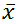, the sample mean, in place of μ, and we use s, the sample standard deviation, in place of σ. Then the value of x looks like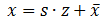Technically, once we make the substitutions, we would really be using a t-distribution of some flavor to model the data. On the other hand, in the example below, since we can get data on every qualified point guard in the NBA as of right now, we can directly compute the mean and standard deviation for the entire population, making this substitution unnecessary in this case. However, students need to be aware of the need for t-procedures.

To show an example of a Normal probability plot, I pulled NBA data from ESPN regarding point guard performance thus far in the 2013-14 regular season. Let's take a look at the top 26 (since there's a tie for 25th place) point guards in the NBA with respect to average points scored per game, the gray column labeled "PTS."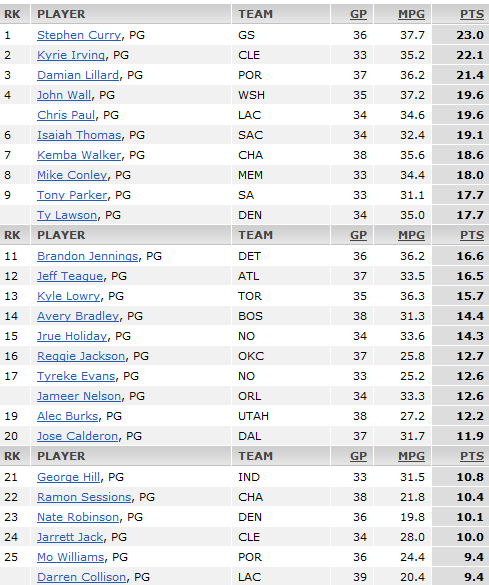Let's enter the data from the table above in the TI-84.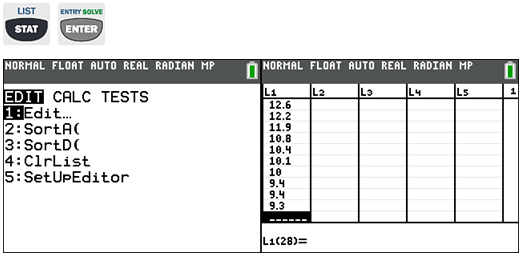Next, let's construct the Normal probability plot for the data.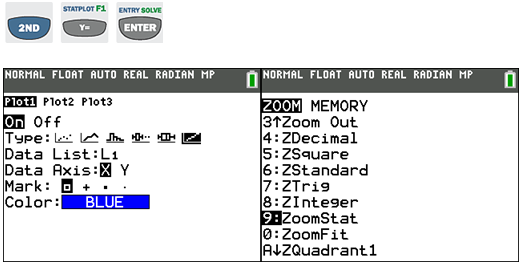So... what exactly does this plot represent? And what makes this representation so important? The x-values obviously correspond to the average points per game value for each point guard. What about the y-coordinate of each point on the graph? The y-coordinate corresponds to the z-score related to that particular x-value. In the screen shot above, Kemba Walker, the point guard with 18.6 points per game, has a z-score of approximately .7039. If the data followed exactly a Normal curve, then all the points on the above graph would lie exactly on a straight line. By looking at the z-score for each data point using this display, we can get a quick insight into whether the data are Normally distributed. Let's look at a boxplot for the same data: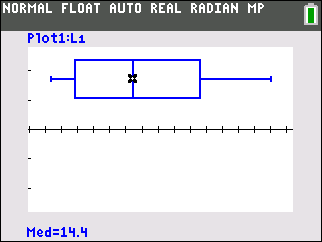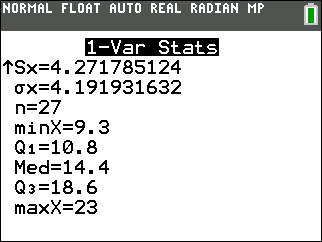We can see, in the plot above, the data for these 26 point guards have no outliers, but there appears to be some skewness. Computing the values (Max - Q3) = 4.4 and (Q1 - Min) = 10.8 - 9.3 = 1.5 and 4.4 > 1.5, we can demonstrate this skewness. This numeric argument doesn't take a lot of calculator kung fu, but we do have to perform an extra computation or two. Looking back at the Normal probability plot, we could use the image to immediately notice the skewness of the data. Suppose we graphed the original z-score equation [z = (x-μ)/σ] on the same graph as the Normal probability plot. In other words, we will make the Normal probability plot. Take a look!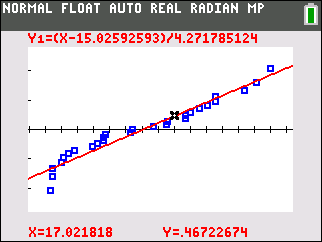We only used 26 data points, so the data is a sample of the population of NBA point guards. Again, if the data were perfectly Normal, all the blue points would be living directly on the red line. We can use our knowledge of linear equations to see clearly what's going on here.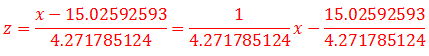So the slope of this red line representing the 'perfectly' Normal data has slope 1/4.271785124. Let's find an equivalent value that's slightly more user friendly: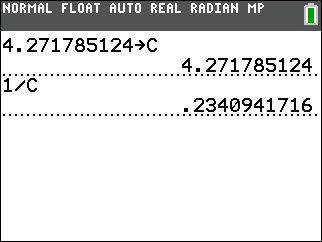If we express this value as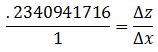notice we can say for every additional unit increase in x, the average points scored per game, we expect to see a z-score increase of .2340941716. Much like when we consider residuals while doing linear regression, when x-values deviate noticeably from the expected red line, they are surprising from the "Normal curve's point of view." The curvature at the left end of the Normal probability plot immediately indicates the skewness of the data. You can find more examples of this on your favorite search engine by asking for "Normal probability plot skewness." If we know how to visually recognize this pattern, we can immediately recognize skewness of data using a Normal probability plot.

This connection between the Normal distribution and why its z-scores are linear has a pretty good explanation on the Wikipedia entry for "Standard score."

This site uses Akismet to reduce spam. Learn how your comment data is processed.